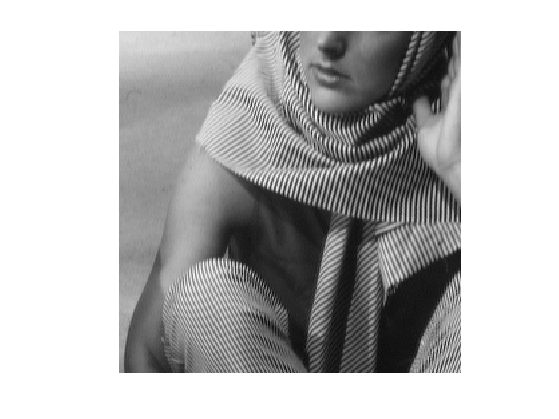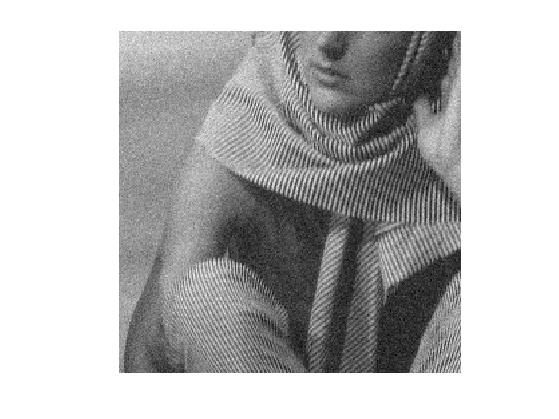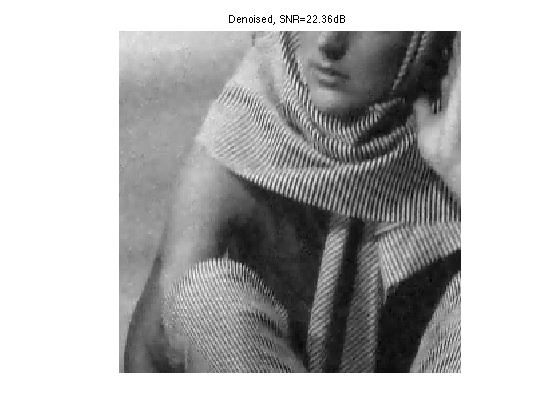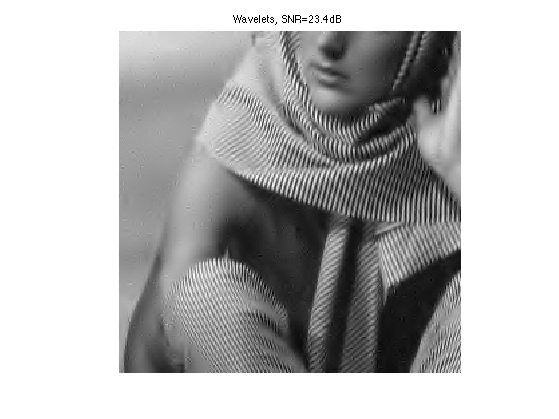$\newcommand{\NN}{\mathbb{N}} \newcommand{\CC}{\mathbb{C}} \newcommand{\GG}{\mathbb{G}} \newcommand{\LL}{\mathbb{L}} \newcommand{\PP}{\mathbb{P}} \newcommand{\QQ}{\mathbb{Q}} \newcommand{\RR}{\mathbb{R}} \newcommand{\VV}{\mathbb{V}} \newcommand{\ZZ}{\mathbb{Z}} \newcommand{\FF}{\mathbb{F}} \newcommand{\KK}{\mathbb{K}} \newcommand{\UU}{\mathbb{U}} \newcommand{\EE}{\mathbb{E}} \newcommand{\Aa}{\mathcal{A}} \newcommand{\Bb}{\mathcal{B}} \newcommand{\Cc}{\mathcal{C}} \newcommand{\Dd}{\mathcal{D}} \newcommand{\Ee}{\mathcal{E}} \newcommand{\Ff}{\mathcal{F}} \newcommand{\Gg}{\mathcal{G}} \newcommand{\Hh}{\mathcal{H}} \newcommand{\Ii}{\mathcal{I}} \newcommand{\Jj}{\mathcal{J}} \newcommand{\Kk}{\mathcal{K}} \newcommand{\Ll}{\mathcal{L}} \newcommand{\Mm}{\mathcal{M}} \newcommand{\Nn}{\mathcal{N}} \newcommand{\Oo}{\mathcal{O}} \newcommand{\Pp}{\mathcal{P}} \newcommand{\Qq}{\mathcal{Q}} \newcommand{\Rr}{\mathcal{R}} \newcommand{\Ss}{\mathcal{S}} \newcommand{\Tt}{\mathcal{T}} \newcommand{\Uu}{\mathcal{U}} \newcommand{\Vv}{\mathcal{V}} \newcommand{\Ww}{\mathcal{W}} \newcommand{\Xx}{\mathcal{X}} \newcommand{\Yy}{\mathcal{Y}} \newcommand{\Zz}{\mathcal{Z}} \newcommand{\al}{\alpha} \newcommand{\la}{\lambda} \newcommand{\ga}{\gamma} \newcommand{\Ga}{\Gamma} \newcommand{\La}{\Lambda} \newcommand{\Si}{\Sigma} \newcommand{\si}{\sigma} \newcommand{\be}{\beta} \newcommand{\de}{\delta} \newcommand{\De}{\Delta} \renewcommand{\phi}{\varphi} \renewcommand{\th}{\theta} \newcommand{\om}{\omega} \newcommand{\Om}{\Omega} \renewcommand{\epsilon}{\varepsilon} \newcommand{\Calpha}{\mathrm{C}^\al} \newcommand{\Cbeta}{\mathrm{C}^\be} \newcommand{\Cal}{\text{C}^\al} \newcommand{\Cdeux}{\text{C}^{2}} \newcommand{\Cun}{\text{C}^{1}} \newcommand{\Calt}{\text{C}^{#1}} \newcommand{\lun}{\ell^1} \newcommand{\ldeux}{\ell^2} \newcommand{\linf}{\ell^\infty} \newcommand{\ldeuxj}{{\ldeux_j}} \newcommand{\Lun}{\text{\upshape L}^1} \newcommand{\Ldeux}{\text{\upshape L}^2} \newcommand{\Lp}{\text{\upshape L}^p} \newcommand{\Lq}{\text{\upshape L}^q} \newcommand{\Linf}{\text{\upshape L}^\infty} \newcommand{\lzero}{\ell^0} \newcommand{\lp}{\ell^p} \renewcommand{\d}{\ins{d}} \newcommand{\Grad}{\text{Grad}} \newcommand{\grad}{\text{grad}} \renewcommand{\div}{\text{div}} \newcommand{\diag}{\text{diag}} \newcommand{\pd}{ \frac{ \partial #1}{\partial #2} } \newcommand{\pdd}{ \frac{ \partial^2 #1}{\partial #2^2} } \newcommand{\dotp}{\langle #1,\,#2\rangle} \newcommand{\norm}{|\!| #1 |\!|} \newcommand{\normi}{\norm{#1}_{\infty}} \newcommand{\normu}{\norm{#1}_{1}} \newcommand{\normz}{\norm{#1}_{0}} \newcommand{\abs}{\vert #1 \vert} \newcommand{\argmin}{\text{argmin}} \newcommand{\argmax}{\text{argmax}} \newcommand{\uargmin}{\underset{#1}{\argmin}\;} \newcommand{\uargmax}{\underset{#1}{\argmax}\;} \newcommand{\umin}{\underset{#1}{\min}\;} \newcommand{\umax}{\underset{#1}{\max}\;} \newcommand{\pa}{\left( #1 \right)} \newcommand{\choice}{ \left\{ \begin{array}{l} #1 \end{array} \right. } \newcommand{\enscond}{ \left\{ #1 \;:\; #2 \right\} } \newcommand{\qandq}{ \quad \text{and} \quad } \newcommand{\qqandqq}{ \qquad \text{and} \qquad } \newcommand{\qifq}{ \quad \text{if} \quad } \newcommand{\qqifqq}{ \qquad \text{if} \qquad } \newcommand{\qwhereq}{ \quad \text{where} \quad } \newcommand{\qqwhereqq}{ \qquad \text{where} \qquad } \newcommand{\qwithq}{ \quad \text{with} \quad } \newcommand{\qqwithqq}{ \qquad \text{with} \qquad } \newcommand{\qforq}{ \quad \text{for} \quad } \newcommand{\qqforqq}{ \qquad \text{for} \qquad } \newcommand{\qqsinceqq}{ \qquad \text{since} \qquad } \newcommand{\qsinceq}{ \quad \text{since} \quad } \newcommand{\qarrq}{\quad\Longrightarrow\quad} \newcommand{\qqarrqq}{\quad\Longrightarrow\quad} \newcommand{\qiffq}{\quad\Longleftrightarrow\quad} \newcommand{\qqiffqq}{\qquad\Longleftrightarrow\qquad} \newcommand{\qsubjq}{ \quad \text{subject to} \quad } \newcommand{\qqsubjqq}{ \qquad \text{subject to} \qquad }$

Dictionary Learning for Denoising

# Dictionary Learning for Denoising

This tour follows the Dictionary Learning numerical tour. It uses a learned dictionary to perform image denoising.

## Installing toolboxes and setting up the path.

You need to download the following files: signal toolbox and general toolbox.

You need to unzip these toolboxes in your working directory, so that you have toolbox_signal and toolbox_general in your directory.

For Scilab user: you must replace the Matlab comment '%' by its Scilab counterpart '//'.

Recommandation: You should create a text file named for instance numericaltour.sce (in Scilab) or numericaltour.m (in Matlab) to write all the Scilab/Matlab command you want to execute. Then, simply run exec('numericaltour.sce'); (in Scilab) or numericaltour; (in Matlab) to run the commands.

Execute this line only if you are using Matlab.

getd = @(p)path(p,path); % scilab users must *not* execute this


Then you can add the toolboxes to the path.

getd('toolbox_signal/');
getd('toolbox_general/');


## Learning the Dictionary

We aim at applying the dictionary learning method to denoising. We thus consider a noisy image obtained by adding Gaussian noise to a clean, unknown image $$f_0 \in \RR^N$$ where $$N=n_0 \times n_0$$.

Noise level $$\sigma$$.

sigma = .06;


Size of the image.

n0 = 256;


name = 'barb';


Display $$f_0$$.

clf;
imageplot(f0);Noisy observations $$f=f_0+w$$ where $$w$$ is a realization of $$\Nn(0,\si^2\text{Id}_N)$$.

f = f0 + sigma*randn(n0);


Display $$f$$.

clf;
imageplot(f);Perform the numerical tour on Dictionary Learning to obtain a dictionary $$D \in \RR^{n \times p}$$

if not(exist('D'))
sparsity_4_dictionary_learning;
end


Width $$w$$ of the patches.

w = sqrt(size(D,1));


Number $$p$$ of atoms.

p = size(D,2);


Dimension $$n= w \times w$$ of the data to be sparse coded.

n = w*w;


## Denoising by Sparse Coding

Here we apply sparse coding in the learned dictionary to the problem of image denoising, as detailed in:

M. Elad and M. Aharon, Image Denoising Via Sparse and Redundant representations over Learned Dictionaries, IEEE Trans. on Image Processing, Vol. 15, no. 12, pp. 3736-3745, December 2006.

The method first extract lots of patches from $$f$$, then perform sparse coding of each patch in $$D$$, and then average the overlapping patch to obtained the denoised image $$f_1$$.

We extract a large number $$m$$ of patches $$Y = (y_j)_{j=1}^m \in \RR^{n \times m}$$ from the noisy image $$f$$.

To extract the patche, we use $y_j(t) = f( a_j + t )$ where $$a_j$$ is the location of patch indexed by $$j$$.

In practice, splitting $$j=(j_1,j_2)$$ as a 2-D index, we use $$a_j = (j_1 q,j_2 q)$$ where $$q>0$$ is an overlap factor ( so that setting $$q=w$$ implies no overlap). Overlap is important to obtain good denoising performance (reduced blocking artifact).

q = 2;


Define regularly space positions for the extraction of patches.

[y,x] = meshgrid(1:q:n0-w/2, 1:q:n0-w/2);
m = size(x(:),1);
Xp = repmat(dX,[1 1 m]) + repmat( reshape(x(:),[1 1 m]), [w w 1]);
Yp = repmat(dY,[1 1 m]) + repmat( reshape(y(:),[1 1 m]), [w w 1]);


Ensure boundary conditions (reflexion).

Xp(Xp>n0) = 2*n0-Xp(Xp>n0);
Yp(Yp>n0) = 2*n0-Yp(Yp>n0);


Extract the $$m$$ patches $$Y$$.

Y = f(Xp+(Yp-1)*n0);
Y = reshape(Y, [n, m]);


Save the mean $$\theta_j$$ of each patch appart, and remove it.

theta = mean(Y);
Y = Y - repmat( theta, [n 1] );


Denoising of the patches is obtained by performing a sparse coding of each patch $$y_j$$ in $$D$$ $\umin{\norm{D x_j - y_j} \leq \epsilon} \norm{x_j}_1.$

The value of $$\epsilon>0$$ is set proportionaly to the noise level $$\sqrt{n}\sigma$$ that contaminates each patch. We denote $$\rho \approx 1$$ the proportionality factor, that is a parameter of the method.

rho = .95;
epsilon = rho*sqrt(n)*sigma;


The sparse coding problem can written equivalently in a way that is easier to deal using proximal splitting schemes. We introduce an auxiliary variable $$u=D x \in \RR^n$$ as follow $\umin{ z=(x,u) \in \RR^p \times \RR^n } F(z) + G(z)$ where for $$z=(x,u)$$, one defines $F(x,u) = \norm{x}_1 + \iota_{B_\epsilon(y)}(u) \qwhereq B_\epsilon(y) = \enscond{u}{ \norm{u-y} \leq \epsilon }$ and $G(x,u) = \iota_{\Cc}(x,u) \qwhereq \Cc = \enscond{(x,u)}{ u=D x }.$

Here we included the constraints using characteristic functions $\iota_{A}(z) = \choice{ 0 \qifq z \in A, \\ +\infty \quad \text{otherwise}. }$

## Douglas-Rachford Algorithm

To minimize the sparse coding problem, we make use of a proximal splitting scheme to minimize an energy of the form $$F(z)+G(z)$$. These schemes are adapted to solve structured non-smooth optimization problem.

They basically replace the traditional gradient-descent step (that is not available because neither $$F$$ nor $$G$$ are smooth functionals) by proximal mappings, defined as $\text{Prox}_{\gamma F}(z) = \uargmin{y} \frac{1}{2}\norm{z-y}^2 + \ga F(y)$ (the same definition applies also for $$G$$).

The Douglas-Rachford (DR) algorithm is an iterative scheme to minimize functionals of the form $\umin{z} F(z) + G(z)$ where $$F$$ and $$G$$ are convex functions for which one is able to comptue the proximal mappings $$\text{Prox}_{\gamma F}$$ and $$\text{Prox}_{\gamma G}$$.

The important point is that $$F$$ and $$G$$ do not need to be smooth. One onely needs then to be "proximable".

A DR iteration reads $\tilde z_{k+1} = \pa{1-\frac{\mu}{2}} \tilde z_k + \frac{\mu}{2} \text{rPox}_{\gamma G}( \text{rProx}_{\gamma F}(\tilde z_k) ) \qandq z_{k+1} = \text{Prox}_{\gamma F}(\tilde z_{k+1},)$

We have use the following shortcuts: $\text{rProx}_{\gamma F}(z) = 2\text{Prox}_{\gamma F}(z)-z$

It is of course possible to inter-change the roles of $$F$$ and $$G$$, which defines another set of iterations.

One can show that for any value of $$\gamma>0$$, any $$0 < \mu < 2$$, and any $$\tilde z_0$$, $$z_k \rightarrow z^\star$$ which is a minimizer of $$F+G$$.

Please note that it is actually $$z_k$$ that converges, and not $$\tilde z_k$$.

Proximal Splitting Methods in Signal Processing, Patrick L. Combettes and Jean-Christophe Pesquet, in: Fixed-Point Algorithms for Inverse Problems in Science and Engineering, New York: Springer-Verlag, 2010.

## Douglas-Rachford for Sparse Coding

In the special case of the constrained sparse coding problem, the proximal mapping of $$G$$ is the orthogonal projection on the convex set $$\Cc$$: $(\tilde x, \tilde u) = \text{Prox}_{\ga G}(x,u) = \text{Proj}_\Cc(x,u).$

It can be computed by solving a linear system of equations since $\tilde u = D \tilde x \qwhereq \tilde x = (\text{Id} + D^* D)^{-1}(f + D^* u).$

Define Proj$$_\Cc$$, by pre-compuring the inverse of $$\text{Id} + D^* D$$.

U = (eye(p) + D'*D)^(-1);
Replicate = @(z)deal(z, D*z);
ProjC = @(x,u)Replicate( U*( x + D'*u ) );


One has $$\text{Prox}_{\ga G} = \text{Proj}_{\Cc}$$, whatever the value of $$\ga$$.

ProxG = @(f,u,gamma)ProjC(f,u);


Function $$F(x,u)$$ is actully a separable sum of a function that only depends on $$x$$ and a function that depends only on $$u$$: $F(x,u) = \iota_{B_\epsilon(y)}(u) + \norm{x}_1.$ The proximal operator of $$F$$ reads $\text{Prox}_{\ga F}(x,u) = ( \text{Proj}_{B_\epsilon(y)}(u), \text{Prox}_{\ga \norm{\cdot}_1 }(x) ).$

In order to speed up the implementation, the DR algorithm will be performed in parallel on all the $$x_j$$. We thus define $$y=Y$$ to be the set of all the patches.

y = Y(:,1:m);


Define the projector $\text{Proj}_{B_\epsilon(y)}(u) = y + (u-y) \max\pa{1 , \frac{\epsilon}{\norm{u-y}} }$

amplitude = @(a)repmat( sqrt(sum(a.^2,1)), [n 1] );
ProjB = @(u)y + (u-y) .* min(1, epsilon./amplitude(u-y) );


The proximal operator of the $$\ell^1$$ norm $$\norm{\cdot}_1$$ is a soft thresholding: $\text{Prox}_{\ga \norm{\cdot}_1}(x)_i = \max\pa{ 0, \frac{\ga}{\abs{x_i}} } x_i.$

ProxL1 = @(x,gamma)max(0,1-gamma./max(1e-9, abs(x))) .* x;


Define the proximal operator of $$F$$.

ProxF = @(x,u,gamma)deal( ProxL1(x,gamma), ProjB(u) );


Set the value of $$\mu$$ and $$\gamma$$. You might consider using your own value to speed up the convergence.

mu = 1;
gamma = 1;


Number of iterations.

niter = 800;


Exercice 1: (check the solution) Implement the DR iterative algorithm on niter iterations. Keep track of the evolution of the minimized energy $$\norm{x}_1$$ during the iterations.

exo1;## Patch Averaging

Once each $$x_j$$ is computed, one obtains the denoised patch $$\tilde y_j = D x_j$$. These denoised patches are then aggregated together to obtained the denoised image: $f_1(t) = \frac{1}{W_t} \sum_j \tilde y_j(t-a_j)$ where $$W_t$$ is the number of patches that overlap at a given pixel location $$t$$ (note that in this formula, we assumed $$\tilde y_j(t-a_j)=0$$ when $$t-a_j$$ falls outside the patch limits).

Approximated patches $$Y_1 = (\tilde y_j)_j = D X$$.

Y1 = reshape(D*x, [w w m]);


Insert back the mean.

Y1 = Y1 - repmat( mean(mean(Y1)), [w w] );
Y1 = Y1 + reshape(repmat( theta, [n 1] ), [w w m]);


To obtain the denoising, we average the value of the approximated patches $$Y_1$$ that overlap.

W = zeros(n0,n0);
f1 = zeros(n0,n0);
for i=1:m
x = Xp(:,:,i); y = Yp(:,:,i);
f1(x+(y-1)*n0) = f1(x+(y-1)*n0) + Y1(:,:,i);
W(x+(y-1)*n0) = W(x+(y-1)*n0) + 1;
end
f1 = f1 ./ W;


Display the result.

clf;
imageplot(clamp(f1), ['Denoised, SNR=' num2str(snr(f0,f1),4) 'dB']);Exercice 2: (check the solution) Compare the obtained result with translation invariant wavelet hard thresholding.

exo2;Exercice 3: (check the solution) Study the influence of the parameter $$\rho$$ on the quality of the denoising. Study the influence of the number $$p$$ of atoms.

exo3;